# Models for bulk material¶

The nanoindentation (or instrumented or depth sensing indentation) is a variety of indentation hardness tests applied to small volumes. During nanoindentation, an indenter is brought into contact with a sample and mechanically loaded.

The following parts give a short overview of models existing in the literature used for the extraction of mechanical properties of homogeneous bulk materials from indentation experiments with geometrically self-similar indenters (conical or sharp).

Please look at the ISO standard (ISO 14577 - 1 to 3), to perform nanoindentation tests on bulk material.

Some authors overviewed/reviewed already the nanoindentation technique :

## Nanoindentation tests on bulk material¶

### Conical and geometrically similar indenters¶

The geometric properties of conical and geometrically similar (Berkovich, Vickers, …) indenters are well described in .

Table 1 Geometric properties of conical indenters.
Indenter Berkovich Vickers Cube-corner Knoop Conical
Shape 3-sided pyramid 4-sided pyramid 3-sided pyramid 4-sided pyramid Conical (angle $$\psi$$)
Semi-angle from the apex 65.3° 68° 35.2644° 86.25° / 65°
Equivalent cone angle 70.32° 70.2996° 42.28° 77.64° $$\psi$$
Projected Area $$24.56h_\text{c}^2$$ $$24.504h_\text{c}^2$$ $$2.5981h_\text{c}^2$$ $$108.21h_\text{c}^2$$ $$\pi{a_\text{c}}^2$$
Volume-depth relation $$8.1873h^3$$ $$8.1681h^3$$ $$0.8657h^3$$
Projected area/face area $$0.908$$ $$0.927$$ $$0.5774$$
Contact radius $$htan\psi$$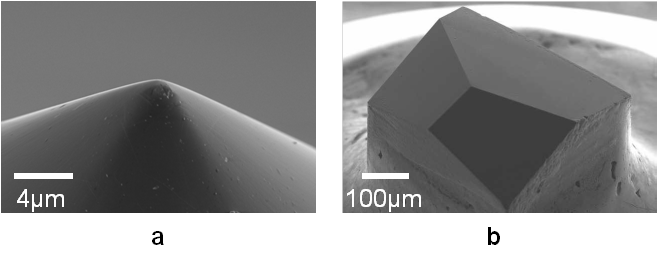Figure 9 a) Conical indenter (45°) and b) Berkovich indenter.

With $$h_\text{c}$$ the contact depth.

These indenters have self-similar geometries which implies a constant strain during indentation and similarity of the stress fields.

Note

Indenters are mainly in diamond. Diamond has a Young’s modulus of 1070GPa and a Poisson’s ratio of 0.07 .

Find here the Matlab function to plot the projected ared of 3-sided pyramidal indenter as a function of indentation depth : projectedArea_3sidePyramid.m.

Find here the Matlab function to plot the the projected ared of 4-sided pyramidal indenter as a function of indentation depth : projectedArea_4sidePyramid.m.

Practically, perfect conical indenters (no tip defect) don’t really exist and they are usually defined as cono-spherical indenters with a tip defect $$h_\text{tip}$$ (see following scheme) .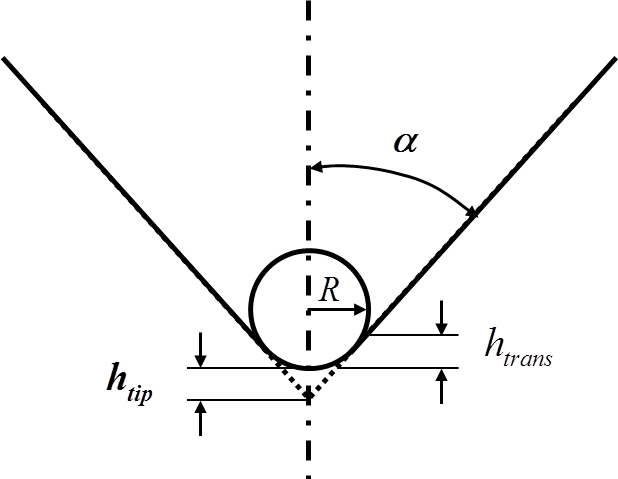Figure 10 Scheme of a cono-spherical indenter.

The radius $$R$$ of the spherical part is calculated from the tip defect $$h_\text{tip}$$ and the cone angle $$\alpha$$, using the following equation. For Berkovich, Vickers and cube-corner indenters, the equivalent cone angle is used to set the cone angle.

(1)$R = \frac{h_\text{tip}}{\frac{1}{sin(\alpha)} - 1}$

In case of a tip defect of 5nm for a Berkovich indenter, a tip radius of $$R = 148\text{nm}$$ is calculated.

The transition depth $$h_\text{trans}$$ between the spherical and the conical parts of a cono-spherical indenter, is calculated from the followig equation:

(2)$h_\text{trans} = R(1-sin(\alpha))$

Find here the Matlab function to calculate the tip radius: tipRadius.m.

Find here the Matlab function to calculate the transition depth: transitionDepth.m.

Find here the Matlab function to calculate the tip defect: tipDefect.m.

In this first part, only quasistatic (or monotonic) nanoindentation is considered, when a load is applied and removed to a sample. Parameters such as contact load $$F_\text{c}$$ (in $$\text{N}$$) and depth of penetration (displacement) $$h_0$$ (in $$\text{m}$$) are continuously recorded at a rapid rate (normally $$10\text{Hz}$$) during loading and unloading steps of the indentation test. Usually, the depth resolution is around the $$\text{nm}$$-level and the load resolution is around $$\text{nN}$$-level.

#### Initial penetration¶

The first correction step in nanoindentation testing is the determination of the initial contact point between the indenter and the sample .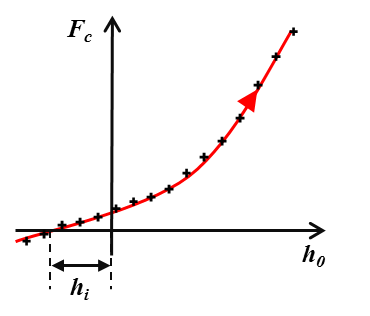Figure 11 Schematic of the estimation of initial point.

Usually, the point of contact is determined from the load-displacement curve, when a sharp rise in the force signal is observed. Then, initial penetration $$h_\text{i}$$ is estimated by extrapolating the recorded load–displacement data back to zero load.

(3)$h = h_0 + h_\text{i}$

With $$h$$ the corrected penetration and $$h_0$$ the recorded penetration.

A schematic of the load-displacement curve obtained from nanoindentation experiment after this first correction is given Figure 12.

The evolution of this curve depends on material properties of the sample and the indenter, and of the indenter’s geometry.

The tangent (or the slope) of the part of the unloading curve at the maximum load gives access to the contact stiffness $$S$$ (in $$\text{N/m}$$):

(4)$S = \frac{dF_\text{c}}{dh}$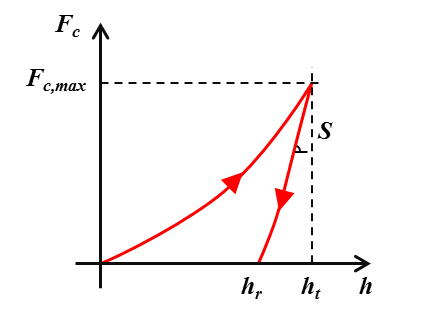Figure 12 Schematic of indentation load-displacement curve.

With $$h_\text{t}$$ the total penetration corrected of the frame compliance and $$h_\text{r}$$ the residual indentation or the plastic depth after unloading .

(5)$h_\text{r} = h - F_\text{c}{S}$

It is worth to mention that for quasistatic nanoindentation, the contact stiffness is a unique value obtained at the maximum load and at the maximum displacement. Nevertheless, it is possible to apply a multiple-point unload method, and then determine the contact stiffness for many indentation depths, as a function of the number of points defined by the user (see Figure 13) .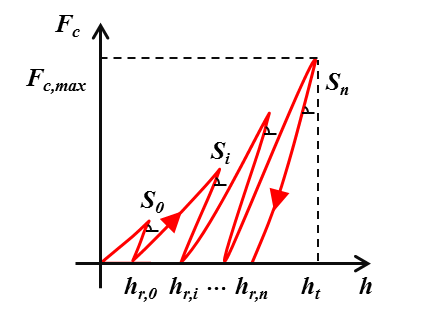Figure 13 Schematic of indentation load-displacement curve with the multiple point unload method (here n points).

#### Frame compliance¶

Before any analysis, it is important to correct raw data of the effect of the frame compliance. The frame compliance is defined by the deflections of the load frame instead of displacement into the studied material. This frame compliance $$C_\text{f}$$ (in $$\text{m/N}$$) contributes to the measured indentation depth and to the contact stiffness .

(6)$h_\text{t} = h - F_\text{c}{C_\text{f}}$
(7)$S = \left(\frac{dh}{dF_\text{c}} - C_\text{f}\right)^{-1}$

To determine the frame compliance, it is required to plot $$\frac{dh}{dF_\text{c}}$$ vs. the corrected total depth $$(1/h_\text{t})$$ or the corrected plastic depth $$(1/{h_\text{c}})$$ (see the following part “Indentation contact topography” for the definition of the plastic depth)  and . Then, a linear fit of this curve gives an intercept with the ordinate axis which is the frame compliance (see Figure 14).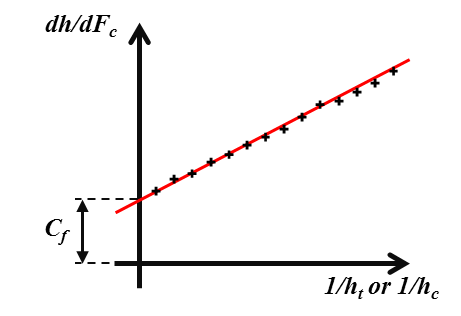Figure 14 Schematic of the plot to determine the frame compliance.

It is advised to perform indentation tests on a variety of bulk standard specimens (fused silica, silicon and sapphire provide a very good range), in order to estimate better the frame compliance.

Moreover, when the sample flexes or has heterogeneities (free edges, interfaces between regions of different properties…), nanoindentation measurements are affected by the structural compliance $$C_\text{s}$$. Then, it is possible to correct experimental data of this artifact by following the experimental approach proposed by .

Loubet et al. founded a good fit to the loading part of the load-displacement curve with a power-law relationship of the form  :

(8)$F_\text{c} = K h_\text{t}^n$

With $$K$$ and $$n$$ constants for a given material for a fixed indenter geometry.

It is possible to find in the litterature sometimes the following equation to fit the loading curve:

(9)$F_\text{c} = K h_\text{t}^n + C_\text{preload}$

With $$C_\text{preload}$$ a constant which is used to account a small preload prior indentation testing .

Using the load-displacement curves analysis performed by Loubet et al., Hainsworth et al. proposed the following relationship to describe loading curves  :

(10)$F_\text{c} = K h_\text{t}^2$

With $$K$$ a constant function of material properties (Young’s modulus and hardness) and the indenter.

In the same time, Giannakopoulos and Larsson established parabolic relationships between the load and the indentation depth, for purely elastic indentation of bulk materials with ideally Berkovich indenter (11)  and Vickers indenter (12) , by numerical studies.

(11)$F_\text{c} = 2.1891 \left(1-0.21\nu -0.01{\nu}^2 -0.41{\nu}^3 \right) \frac{E}{1-{\nu}^2} h_\text{t}^2$
(12)$F_\text{c} = 2.0746 \left(1-0.1655\nu -0.1737{\nu}^2 -0.1862{\nu}^3 \right) \frac{E}{1-{\nu}^2} h_\text{t}^2$

With $$\nu$$ the Poisson’s ratio and $$E$$ the Young’s modulus of the indented material.

Finally, it is first important to cite the work of Malzbender et al., who developped the relationship between the load and the indentation depth for elastoplastic materials, based on the knowledge of the Young’s modulus and the hardness values of the material . Then, It is worth to mention the model of Oyen et al., who described sharp indentation behavior of time-dependent materials .

Pharr and Bolshakov founded that unloading curves were well described by the following power-law relationship  :

(13)$F_\text{c} = \alpha_\text{u} \left(h_\text{t} - h_\text{r}\right)^m$

Where $$h_\text{r}$$ is the final displacement after complete unloading, and $$\alpha_\text{u}$$ and $$m$$ are material constants. Many experiments performed by Pharr and Bolshakov leaded to an average value for $$m$$ close to $$1.5$$ for the Berkovich indenter.

The mechanical response of a material is function of the imposed indentation strain rate $$\dot{\epsilon}$$ (in $$\text{s}^{-1}$$) . Thus, it is meaningful to perform indentation tests with a constant indentation strain rate.

(14)$\dot{\epsilon} = \frac{\dot{h}}{h} = \frac{1}{2} \frac{\dot{F_\text{c}}}{F_\text{c}}$

### Indentation contact topography¶

The indentation total depth is rarely equal to the indentation contact depth. Two kind of topography can occur:

• the pile-up (indentation contact depth > indentation total depth) (see Figure 15 a and Figure 16);
• the sink-in (indentation contact depth < indentation total depth) (see Figure 15 b).

The flow of material below the indenter is function of mechanical properties of the material.

Pile-up occurs when work-hardening coefficient is low ($$< 0.3$$) or if the ratio yield stress over Young’s modulus is less than $$1%$$ ,  and .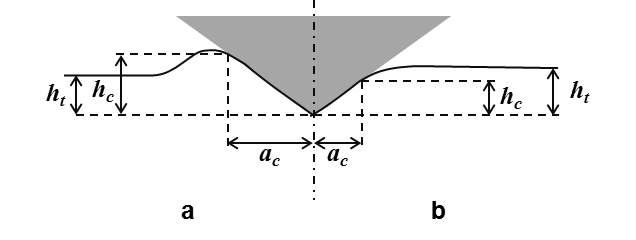Figure 15 Schematic of indentation contact topography : a) “pile-up” and b) “sink-in”.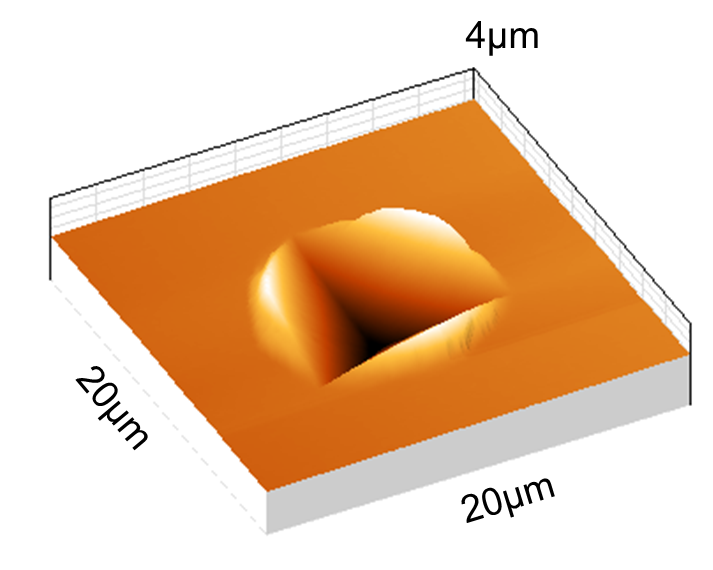Figure 16 Residual topography of a Berkovich indent in PVD Gold thin film (500nm thick) with “pile-up” surrounding the indent, measured by atomic force microscopy.

Three main models defining the depth of contact $$h_\text{c}$$ were developed to take into account this indentation contact topography.

(15)$h_\text{c} = h_\text{t} - \frac{F_\text{c}}{S}$

Model of Oliver and Pharr ,  and  in case of sink-in:

(16)$h_\text{c} = h_\text{t} - \epsilon \frac{F_\text{c}}{S}$

Where $$\epsilon$$ is a function of the indenter’s geometry ($$0.72$$ for conical indenter, $$0.75$$ for paraboloids of revolution and $$1$$ for a flat cylindrical punch). An expression of $$\epsilon$$ as a function of the power law exponent $$m$$ of the unloading curve fit has been proposed by Pharr et Bolshakov  :

$\epsilon = m \left( 1 - \frac{ 2 \Gamma \left( \frac{m}{2(m-1)} \right)} {\sqrt[]{\pi} \Gamma \left( \frac{1}{2(m-1)}(m-1) \right)} \right)$

With $$\Gamma$$ a Matlab function which interpolates the factorial function : gamma.

Find here the Matlab function to plot the $$\epsilon$$ function : epsilon_oliver_pharr.m.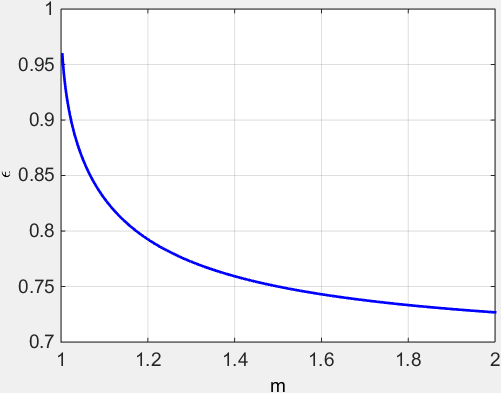Figure 17 Evolution of epsilon as a function of the power law exponent m of the unloading curve.

$$0.72$$ should be most applicable for a Berkovich indenter, which is more like a cone than a paraboloid of revolution. But, Oliver and Pharr concluded after a large number of experiments that the best value for the Berkovich indenter is $$0.75$$.

More recently, Merle et al. have found experimentally with indentation test in fused silica, a value of $$0.76$$ for $$\epsilon$$, which is in a good agreement with the literature for a paraboloid of revolution .

Model of Loubet et al. ,  in case of pile-up:

(17)$h_\text{c} = \alpha \left(h_\text{t} - \frac{F_\text{c}}{S} + h_0\right)$

Where $$\alpha$$ is a constant function of the indented material (usually around $$1.2$$) and the tip-defect $$h_0$$.

Knowing the depth of contact, it is possible to determine the area of contact $$A_\text{c}$$ (in $$\text{m}^{2}$$) for a perfect conical indenter (with a semi-angle from the apex $$\theta$$):

(18)$A_\text{c} = \pi h_\text{c}^2 tan^2\left(\theta\right)$

But, because conical indenters present imperfections and Berkovich or Vickers indenters are not perfectly conical, a general formulae of the contact area has been established by Oliver and Pharr ,  :

(19)$A_\text{c} = C_0 h_\text{c}^2 + \sum_{n=1}^8{C_\text{n} h_\text{c}^{1/2^{n-1}}}$

With the coefficients $$C_0$$ and $$C_\text{n}$$ obtained by curve fitting procedures, from nanoindentation experiments in fused silica (amorphous and isotropic material).

For a perfect Berkovich indenter $$C_0$$ is equal to $$24.56$$ and for a perfect Vickers indenter $$C_0$$ is equal to $$24.504$$ (see Table 1).

The second term of the area function $$A_\text{c}$$ describes a paraboloid of revolution, which approximates to a sphere at small penetration depths. A perfect sphere of radius $$R$$ is defined by the first two terms with $$C_0 = -\pi$$ and $$C_1 = 2 \pi R$$. The first two terms also describe a hyperboloid of revolution, a very reasonable shape for a tip-rounded cone or pyramid that approaches a fixed angle at large distances from the tip.

An equivalent contact radius $$a_\text{c}$$ (in $$\text{m}$$) is also defined based on the area function.

(20)$a_\text{c} = \sqrt\frac{A_\text{c}}{\pi}$

One other way to express the function area is that suggested by Loubet et al. , which describes a pyramid with a small flat region on its tip, the so-called tip defect ($$h_0$$). This geometry is described by the addition of a constant to the first two terms in (19).

Find here the Matlab function to calculate the contact depth, the function area and the contact radius: model_function_area.m.

Recently, in the paper of Yetna N’jock M. et al. , a criterion was proposed to forecast the behaviour during indentation experiments, following Giannakopoulos and Suresh methodology . After analyzing either Vickers or Berkovich indentation tests on a wide range of materials, the following criterion is established $$\Delta$$ :

(21)$\Delta = \frac{h_\text{r}^{'}}{h_\text{t}^{'}}$

With $$h_\text{r}^{'}$$ and $$h_\text{t}^{'}$$ residual contact depth and maximum depth after applying a compliance correction. Three preponderant deformation modes are distinguished :

• $$\Delta = 0.83$$ no deformation mode is preponderant;
• $$\Delta < 0.83$$ implies sink-in formation;
• $$\Delta > 0.83$$ implies pile-up formation.

Giannakopoulos and Suresh founded a critical value for a similar criterion about $$0.875$$ .

## Dynamic nanoindentation¶

The dynamic indentation is when a small dynamic oscillation (usually $$2\text{nm}$$ of amplitude) with a given frequency ($$\omega$$) (usually $$45\text{Hz}$$) is imposed on the force (or displacement) signal. The amplitude of the displacement (or load) and the phase angle between the force and displacement signals ($$\phi$$) are measured using a frequency-specific amplifier ,  and . This technique allows to calculate the elastic stiffness and so the elastic properties continuously during the loading of the indenter , . This technique is named Continuous Stiffness Measurement (CSM) for Agilent - MTS nanoindenter and Dynamic Mechanical Analysis (DMA) (using the CMX control algorithms) for Hysitron nanoindenter.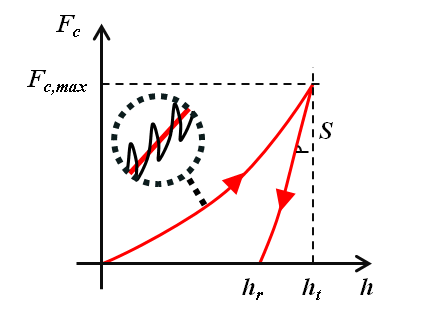Figure 18 Schematic of the dynamic loading cycle.

(22)$S = \left[\frac{1}{\frac{F_\text{c}}{h_\text{t}}\cos\phi-\left(K_\text{s} - m\omega^2\right)} - \frac{1}{K_\text{f}}\right]^{-1}$
(23)$C\omega = \frac{F_\text{c}}{h_\text{t}}\sin\phi - C_\text{s}\omega$

With $$m$$ the mass of the indenter column, $$C$$ the harmonic contact damping in $$\text{N.s/m}$$, $$C_\text{s}$$ the system damping coefficient in $$\text{N.s/m}$$, $$K_\text{s}$$ the stiffness of the indenter support springs in $$\text{N/m}$$ and $$K_\text{f}$$ the stiffness of the load frame in $$\text{N/m}$$. $$C\omega$$ and C_text{s}omega represent respectively the tip–sample damping factor and the system damping coefficient.

Values $$m$$, $$C_\text{s}$$, $$K_\text{s}$$ and $$K_\text{f}$$ are function of the equipment used and are determined during calibration process.

This solution allows to determine the material properties as a continuous function of the indentation depth, but Pharr et al. have highlighted the influence of displacement oscillation on the basic measured quantities . According to the authors, “the sources of the measurement error have their origin in the relative stiffness of the contact and its relation to the displacements that can be recovered during the unloading portion of the oscillation”. Based on that, the authors proposed the following corrections to determine the actual load ($$F_\text{c,act}$$), the actual displacement ($$h_\text{t,act}$$) and the actual stiffness ($$S_\text{act}$$):

(24)$F_\text{c,act} = F_\text{c} + \frac{\Delta F_\text{c}}{2} = F_\text{c} + \sqrt{2}{\Delta F_\text{c,rms}}$
(25)$h_\text{t,act} = h_\text{t} + \frac{\Delta h_\text{t}}{2} = h_\text{t} + \sqrt{2} \Delta h_\text{t,rms}$
(26)$S_\text{act} = \frac{1}{\sqrt{2\pi}} \left(\frac{1}{K}\right)^\frac{1}{m} \left[1-\left(1-S{\frac{2\sqrt{2}{\Delta h_\text{t,rms}}} {F_\text{c,max}}}\right)^\frac{1}{m}\right]\frac{F_\text{c,max}}{{\Delta h_\text{t,rms}}}$

With $$K$$ and $$m$$ constants determined from unloading curves. These constants are related by the following equation:

(27)$K = \left(\frac{2}{{m\sqrt{\pi}}}\right)^m$

Pharr and Bolshakov founded a value of $$1.380$$ for $$m$$ after many Berkovich indentation tests on a variety of materials . Thus, a value of $$0.757$$ is used for the constant $$K$$, using (27).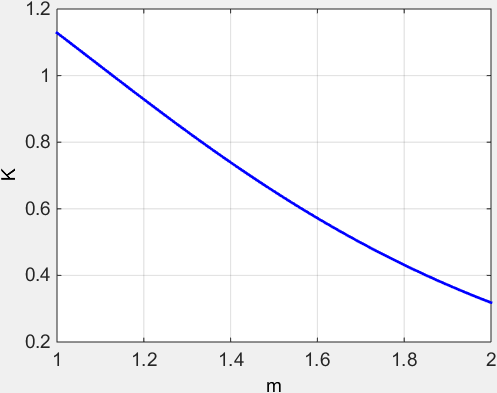Figure 19 Evolution of K as a function of m.

Find here the Matlab function to calculate the corrections to apply on depth, load and stiffness during dynamic nanoindentation: CSM_correction.m.

Find here the Matlab function to calculate the constant $$K$$ as a function of $$m$$: unload_k_m.m.

## Extraction of elastic properties¶

Bulychev et al.  and Shorshorov M. K. et al.  were the first to determine the reduced Young’s modulus (or elastic modulus or storage modulus) of a material with the relationships established by Love , Galin  and Sneddon , between the applied load and the displacement during an indentation test of an elastic material.

They proposed to expressed the reduced Young’s modulus $$E^{*}$$ (in $$\text{GPa} = \text{N/m}^2$$) as a function of the contact area and the contact stiffness:

(28)$E^{*} = \frac{1}{2} \sqrt\frac{\pi}{A} S$

Then, Oliver and Pharr ,  democratized this formulae after introducing a correction factor identified by King :

(29)$E^{*} = \frac{1}{{2\beta}} \sqrt\frac{\pi}{A} S$

With $$\beta$$ a geometrical correction factor equal to :

• $$1$$ for circular indenters (e.g.: conical and spherical indenter);
• $$1.034$$ for three-sided pyramid indenters (e.g.: Berkovich indenter);
• $$1.012$$ for four-sided pyramid indenters (e.g.: Vickers indenter).

Woirgard has demonstrated analytically that the exact value of $$\beta$$ for the perfectly sharp Berkovich indenter should be $$1.062$$ .

Some authors proposed another correction factor function of the angle of the conical indenter and the Poisson’s ratio of the indented material  and . For a conical indenter with an half-angle of $$\gamma \leq 60^{\circ}$$ (e.g.: Cube-Corner indenter), the analytical approximation is:

(30)$\beta = 1 + \frac{{\left(1-2\nu\right)}}{{4\left(1-\nu\right)tan\gamma}}$

For a conical indenter with larger half-angle (e.g.: Berkovich indenter), the analytical approximation is:

(31)$\beta = \pi{ \frac{\pi/4 + 0.1548cot\gamma{\frac{1-2\nu}{4\left(1-\nu\right)}}} {\left[\pi/2-0.8311cot\gamma{\frac{1-2\nu}{4\left(1-\nu\right)}}\right]^2}}$

With $$\nu$$ the Poisson’s ratio of the indented material.

Find here the Matlab function to plot the $$\beta$$ function of Hay et al.: beta_hay.m.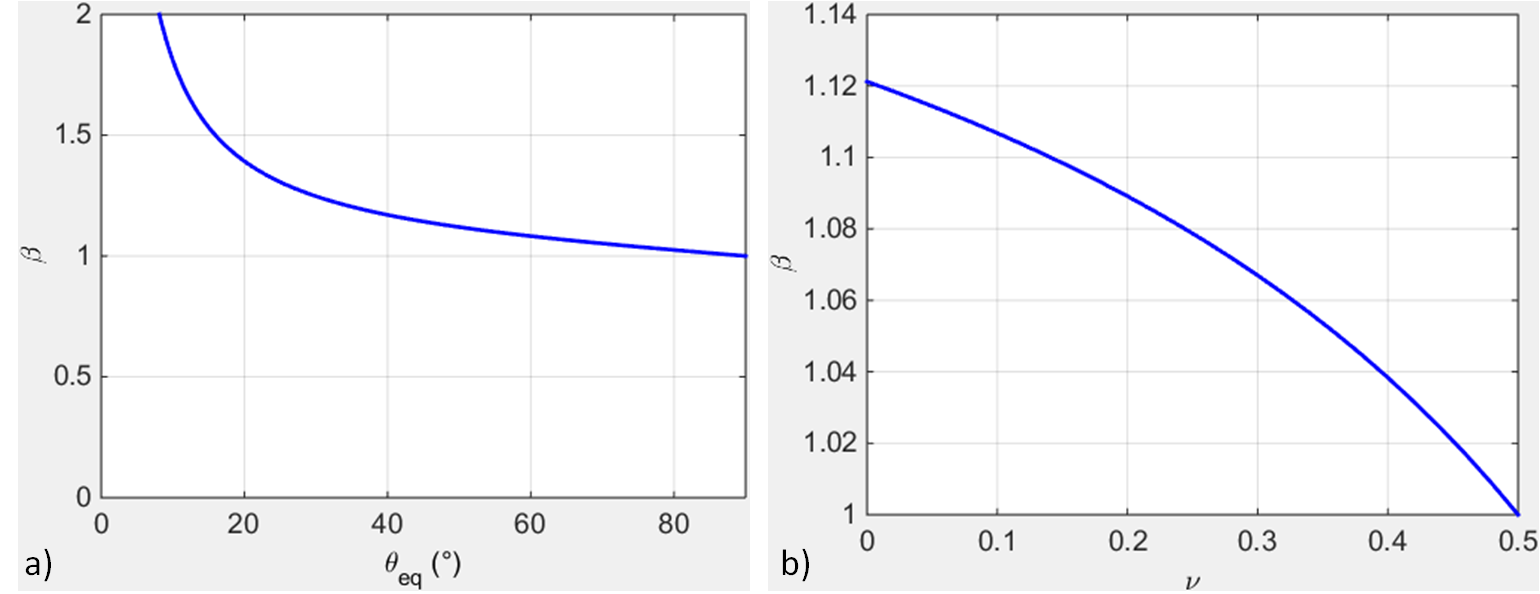Figure 20 Plots of beta Hay : a) as a function of the half-angle of the conical indenter (for a Poisson’s ratio of 0.3), and b) as a function of the Poisson’s ratio for a Berkovich indenter.

Knowing the material properties of the indenter, it is possible to calculate the reduced Young’s modulus $$E^{'}$$ (in $$\text{GPa} = \text{N/m}^2$$) of the indented material.

(32)$\frac{1}{E^{'}} = \frac{1}{E^{*}} - \frac{1}{E_\text{i}^{'}}$
(33)$E = E^{'} \left(1-\nu^{2}\right)$
(34)$E_\text{i}^{'} = \frac{E_\text{i}}{\left(1-\nu_\text{i}^{2}\right)}$

With $$\nu$$ the Poisson’s ratio of the indented material and $$\nu_\text{i}$$ the Poisson’s ratio of the material of the indenter.

Note

This method used to analyze indentation data is based on equations valid for isotropic homogeneous elastic solids.

Find here the Matlab function to calculate the Young’s modulus: model_elastic.m.

## Extraction of viscoelastic properties¶

Viscoelasticity of a material, can be characterized locally by nanoindentation using CSM or DMA techniques ,  and . This technique also called nano-DMA is a suitable technique for mechanical characterization of polymers and is complementary to traditional macroscale DMA and ThermoMechanical Analysis (TMA).

If dynamic nanoindentation is performed, a sinusoidal input is applied and the output signal is monitored. But, in case of a linear viscoelastic material, the output signal, which is still sinusoidal, can lag the input signal, and it is convenient to express the overall constitutive behavior in terms of the complex modulus given by:

(35)$E^{*} = E^{'*} + iE^{''*}$

with $$E^{'*}$$ the reduced storage modulus and $$E^{''*}$$ the reduced loss modulus (both in $$\text{GPa} = \text{N/m}^2$$), and with $$i^{2} = -1$$. The storage modulus is in phase with the deformation and related to the elastic behavior. The storage modulus is defined using (29). Thus, the reduced loss modulus $$E^{''*}$$ is the out-of-phase component and related to the viscous behavior. $$E^{''*}$$ is characteristic of internal damping (equal to a loss of energy due to internal friction) and defined by the following equation:

(36)$E^{''*} = \frac{C\omega}{2} \sqrt\frac{\pi}{A}$

with $$C\omega$$ the contact damping, given by (23).

It is convinient to calculate the loss tangent ($$tan{\delta}$$) also called the loss factor ($$\eta$$) or phase angle, which is defined by:

(37)$tan{\delta} = \eta = \frac{E^{'*}}{E^{''*}} = \frac{C\omega}{S}$

Find here the Matlab function to calculate the loss modulus: loss_modulus.m.

Find here the Matlab function to calculate the loss tangent or loss factor: loss_tangent.m.

## Extraction of plastic properties¶

The hardness $$H$$ (in $$\text{GPa} = \text{N/m}^2$$) of the material is defined according to Oliver and Pharr , by the following expression:

(38)$H = \frac{F_\text{c,max}}{A_\text{c}}$

Find here the Matlab function to calculate the hardness: model_hardness.m.

## Energy approach¶

Another way to access indentation data is the use of the energy $$W_\text{tot}$$ (in $$\text{J} = \text{N/m}$$) dissipated during the indentation. The elastic $$W_\text{e}$$ and plastic $$W_\text{p}$$ energies are respectively based on the integrals of the loading and unloading curves (see Figure 21)  and .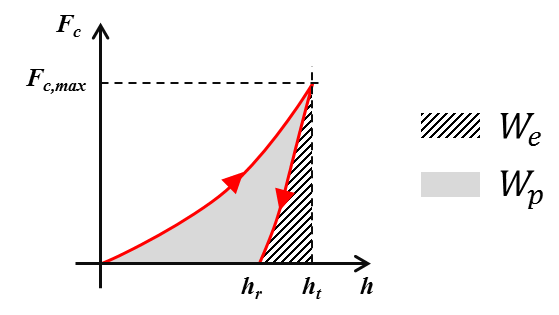Figure 21 Schematic representation of indentation load–displacement curves with definition of different works of indentation.

(39)$W_\text{tot} = \int_{0}^{h_\text{t}} {F_\text{c}\left(dh\right)}$
(40)$W_\text{e} = \int_{h_\text{r}}^{h_\text{t}} {F_\text{c}\left(dh\right)}$
(41)$W_\text{p} = W_\text{tot} - W_\text{e}$

The “trapz” Matlab function is used to calculate the area below the load-displacement curve: trapz.m.

## Indentation recovery index¶

From the load-displacement curve, it is possible to define a parameter known as indentation recovery index ($$\eta_\text{i}$$) . This index is defined by the ratio between recoverable or elastic deformation energy and the total deformation energy:

(42)$\eta_\text{i} = \frac{W_\text{e}}{W_\text{tot}} = \frac{W_\text{tot}-W_\text{p}}{W_\text{tot}} = \frac{h_\text{t}-h_\text{r}}{h_\text{t}}$

Higher value of the indentation recovery index indicates greater capability to accommodate deformation during indentation test.

Find here the Matlab function to calculate the indentation recovery index : recovery_index.m.

## Methodology to extract properties without the function area¶

The ratio of the irreversible work $$W_\text{tot} - W_\text{e}$$ to the total work $$W_\text{tot}$$, appears to be a unique function of the Young’s modulus and the hardness of the material, independent of the work-hardening behavior .

(43)$\frac{{W_\text{tot} - W_\text{e}}}{W_\text{tot}} = 1 - 5\frac{H}{E^{*}}$

Then, combining the expression of the reduced Young’s modulus (29) with the expression of the hardness (38), leads to the following equation  and :

(44)${\beta}^2 \frac{4}{\pi} \frac{F_\text{c,max}}{S^2} = \frac{H}{{E^{*}}^2}$

The $$\beta$$ is initially not present in the equation given by  or assumed to be equal to 1 in .

These two last equations represent two independent relations that can be solved for $$H$$ and $$E^{*}$$ in a manner that does not directly involve the contact area.

The equation (44) is used as well to determine coefficients of the function area (19). Based on the assumption that the hardness and the Young’s modulus remain constant during indentation test in fused silica (isotropic material), the evolution of the ratio $$\frac{F_\text{c,max}}{S^2}$$ should stay constant as well in function of the indentation depth.

In 2012, Guillonneau et al. proposed a model to extract mechanical properties without using the indentation depth  and . The method is based on the detection of the second harmonic for dynamic indentation testing. This model is interesting especially for penetration depths in the range of $$25$$ to $$100\text{nm}$$, where the uncertainties related to the displacement measurement disturb a lot.

## Methodology to minimize displacement measurement uncertainties using dynamic nanoindentation testing¶

Guillonneau et al. proposed a methodology to minimize displacement measurement uncertainties using dynamic nanoindentation . The following equations are developped using respectively Loubet’s (17) and Oliver and Pharr’s models (16). Hardness and elastic modulus can be calculated independently of the indentation depth and the tip defect.

(45)$h_\text{c} = \alpha\left(h-\frac{H\pi tan(\theta)}{2E^{'*}}h_\text{c}+h_0\right)$
(46)$\frac{dh_\text{c}}{dh} = \frac{\alpha}{1+\frac{\alpha H\pi tan(\theta)}{2E^{'*}}}$

In this expression, $$\frac{dh_\text{c}}{dh}$$ can be determined by a simple linear fit of the plot $$h_\text{c} = f(h)$$. Thus, $$\frac{H}{E^{'*}}$$ can be computed as :

(47)$\frac{H}{E^{'*}} = \frac{2}{\pi tan(\theta)} \left(\frac{1}{\frac{dh_\text{c}}{dh}}-\frac{1}{\alpha} \right)$

Then, $$E^{'*}$$ can be calculated using (44) and $$H$$ knowing $$E^{'*}$$

(48)$E^{'*} = \frac{{S}^2}{2 F_\text{c} tan(\theta)} \left(\frac{1}{\frac{dh_\text{c}}{dh}}-\frac{1}{\alpha} \right)$
(49)$H = \frac{4 F_\text{c} {E^{'*}}^2}{\pi {S}^2}$

(48) and (49) can be extended to the Oliver and Pharr’s contact model (16).

(50)$E^{'*} = \frac{{S}^2}{2 \epsilon F_\text{c} tan(\theta)} \left(\frac{1}{\frac{dh_\text{c}}{dh}}-\frac{1}{\alpha} \right)$
(51)$H = \frac{4 F_\text{c} {E^{'*}}^2}{\pi {S}^2}$

Find here the Matlab function to calculate elastic modulus using Guillonneau’s methodology: model_elasticModulus_Guillonneau.m.

Find here the Matlab function to calculate hardness using Guillonneau’s methodology : model_hardness_Guillonneau.m.